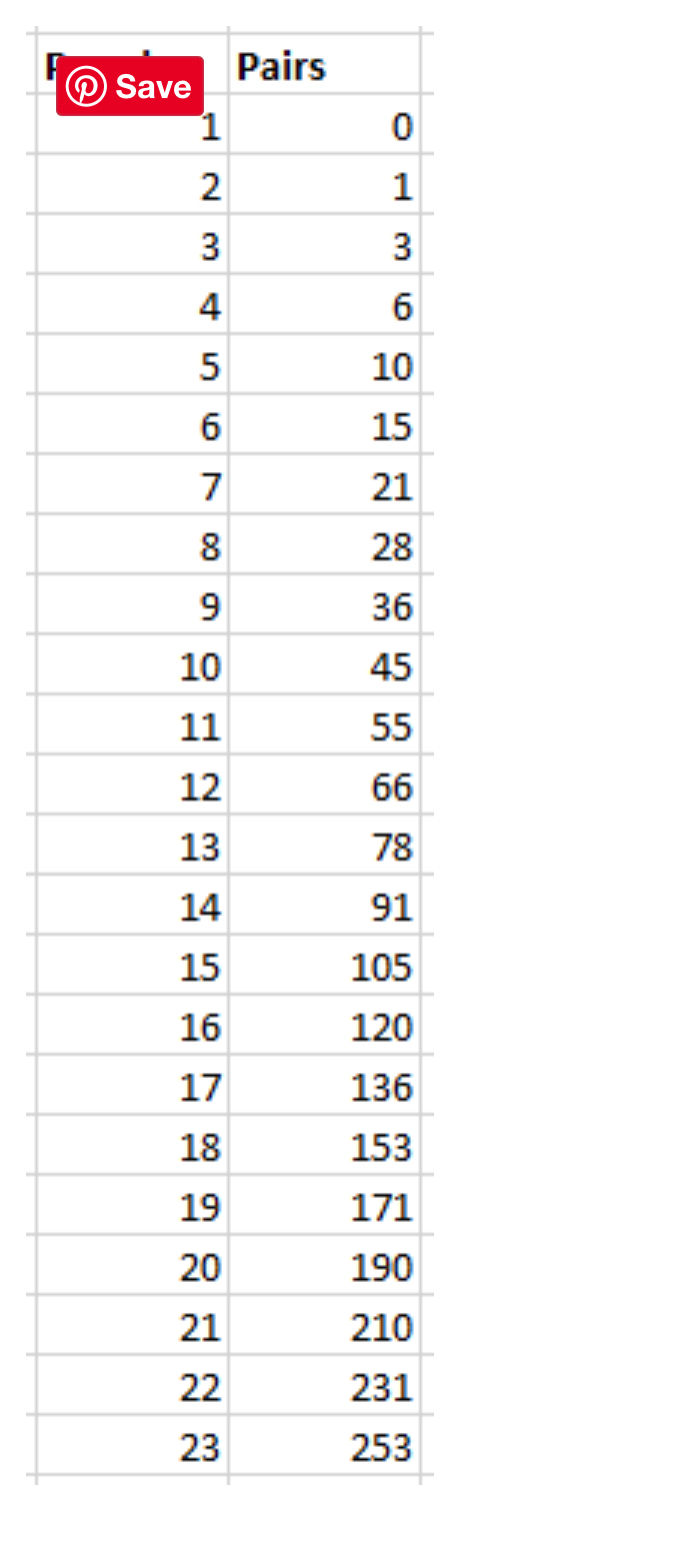top of page
Search
• Lucian@going2paris.net

# Dang! Wrong Again. The Birthday Problem.

Charlottesville

April 7, 2023

A classic example of where my mind's System 1 thinks the answer is easy and doesn't call on lazy but rational System 2 to solve the problem!!

The Birthday Problem in statistics asks, how many people do you need in a group to have a 50% chance that at least two people will share a birthday? Go ahead and think about that for a moment. The answer surprises many people. We’ll get to that shortly.

In this post, I’ll not only answer the birthday paradox, but I’ll also show you how to calculate the probabilities for any size group, run a computer simulation of it, and explain why the answer to the Birthday Problem is so surprising.

Calculating Probabilities for the Birthday Problem

Many people guess 183 because that is half of all possible birthdays, which seems intuitive.

Unfortunately, intuition doesn’t work well for solving this problem. So, let’s get straight to calculating probabilities for people sharing birthdays.

For these calculations, we’ll make a few assumptions. First, we’ll disregard leap year. That simplifies the math, and it doesn’t change the results by much. We’ll also assume that all birthdays have an equal probability of occurring.

Let’s start with one person, and then add people in one at a time to illustrate how the calculations work. For these calculations, it is easier to calculate the probability that no one shares a birthday. We’ll then take that probability and subtract if from one to derive the probability that at least two people share a birthday.

1 – Probability of no match = Probability of at least one match

For the first person, there are no birthdays already covered, which means that there is a 365/365 chance that there is not a shared birthday. That makes sense. We have just one person.

1 - {\displaystyle \left( \frac {365}{365}\right)} = 0

Now, let’s add in the second person. The first person covers one possible birthday, so the second person has a 364/365 chance of not sharing the same day. We need to multiply the probabilities of the first two people and subtract from one.

1 - (365/365)(364/365)= 0.0027

For the third person, the previous two people cover two dates. Hence, the third person has a probability of 363/365 for not sharing a birthday.

1-(365/365)(364/365)(363/365) = 0.0082

Now, you’re seeing the pattern for how to calculate the probability for a given number of people. Here’s the general form of the equation:Graphing the Birthday Problem Probabilities

Using Excel, I can calculate and graph the probabilities for any size group.By assessing the probabilities, the answer to the Birthday Problem is that you need a group of 23 people to have a 50.73% chance of people sharing a birthday!

Most people don’t expect the group to be that small. Also, notice on the chart that a group of 57 has a probability of 0.99. It’s virtually guaranteed!

Don’t worry. I’ll get to explaining this surprising result shortly.

Why is the Group Size So Small for the Birthday Problem?

Like the Monty Hall Problem, most people think the answer to the Birthday Problem is surprising and it hurts their brain a bit! However, the answer is entirely correct, Let’s examine why the answer is counterintuitive.

Often people will think of their birthday and the probability that someone will match that specific date. However, the problem asks about any two individuals sharing a birthday. That means you have to compare all possible pairs of individuals.

Assessing all pairs causes the number of comparisons to increase rapidly—and therein lies the source of confusion.

The formula for the number of comparisons between pairs of N people is: (N*(N-1))/2. As you can see in the table below, the number comparisons snowballs to 253 for only 23 people!For sharing a birthday, each pair has a fixed probability of 0.0027 for matching. That’s low for just one pair. However, as the number of pairs increases rapidly, so does the probability of a match. With 23 people, you need to compare 253 pairs. With that many comparisons, it becomes difficult for none of the birthday pairs to match.

When there are 57 people, there are 1,596 pairs to compare, and it’s virtually guaranteed with a 0.99 probability that at least one pair will match birthdays.

I love problems like this where intuition leads you astray but math saves the day!